# Two-constants theorem

Letbe a finitely-connected Jordan domain in the-plane and let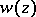be a regular analytic function insatisfying the inequality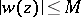, as well as the relationon some arcof the boundary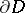. Then, at each pointof the set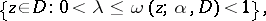where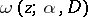is the harmonic measure of the arcwith respect toat, the inequality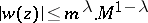is satisfied. If for some(satisfying the condition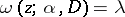) equality is attained, equality will hold for alland for all,, while the functionin this case has the form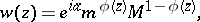whereis a real number and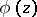is an analytic function infor which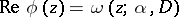, .

The two-constants theorem gives a quantitative expression of the unique determination of analytic functions by their boundary values and has important applications in the theory of functions . Hadamard's three-circles theorem (cf. Hadamard theorem) is obtained from it as a special case. For possible analogues of the two-constants theorem for harmonic functions in space see , .